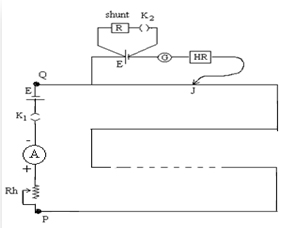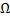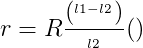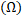The Potentiometer-Internal resistance of a cell

# Materials Required:

• Potentiometer
• Battery eliminator
• Two one way keys
• Rheostat of low resistance
• Galvanometer
• Two resistance boxes
• Leclanche cell
• Jockey
• Ammeter
• Connecting wires

# Real lab Procedure• Arrange the required materials on a table and make the connections as per the connection diagram.
• Tight the plugs of the resistance box.
• To test the connection, insert the key k1 and note the ammeter reading. Introduce a sufficiently high resistance on the resistance box (H.R). Place the jockey at the two end points of the wire. If the galvanometer shows opposite deflection, then the connections are correct.
• Without inserting the key k2, slide the jockey along the potentiometer wire and stops when null point is obtained.
• Measure the balancing length l1 between this point and the end P of the potentiometer.
• Now, introduce plugs in keys k1 and k2. Take out a small resistance from the resistance box R connected in parallel with the cell.
• Again slide the jockey along the potentiometer wire to obtain the null point. Measure the new balancing length l2 based on this point.
• Reduce the value of R successively and in each time, measure the balancing length.
• Keep the reading on the ammeter constant throughout the observation.

# Simulator Procedure (as performed through the Online Labs)

• Select the primary cell form the drop down list.
• Select the rheostat resistance using the slider.
• To see the circuit diagram, click on the ‘Show circuit diagram’ check box seen inside the simulator window.
• Connections can be made as seen in the circuit diagram by clicking and dragging the mouse form one connecting terminal to the other connecting terminal of the devices to be connected.
• Once all connections are made, click and drag the key 1 to insert it into the switch.
• The ammeter shows a deflection.
• To check whether the connections are correct or not, drag the jockey and place it at the two end points of the wire. If the galvanometer shows opposite deflections, the connections are correct.
• To obtain the null point slide the jockey along the potentiometer wire.
• You can see the first balancing length in the popup.
• Select the desired shunt resistance from the resistance box.
• Click and drag the key 2 to insert it into the switch.
• Again slide the jockey along the potentiometer wire to obtain the null point.
• You can see the second balancing length in the popup.
• You can calculate the internal resistance for each set of balancing lengths.
• To verify your result click on the ‘Show result’ check box.
• To redo the experiment, click on the ‘Reset’ button.

# Observations:

 Sl.No. Resistance, RPosition of null point (cm) Internal resistanceWithout shunt R, l1 (cm) With shunt R, l2 (cm) 1 2 3 4 5

# Calculations

Calculate the value of r for each set of l1 and l2. The mean of the calculated values gives the internal resistance (r) of the given cell.

# Result:

The internal resistance of the given primary cell, r =  ..……….. Ω.

Cite in Scientific Research:

Nedungadi P., Raman R. & McGregor M. (2013, October). Enhanced STEM learning with Online Labs: Empirical study comparing physical labs, tablets and desktops. In Frontiers in Education Conference, 2013 IEEE (pp. 1585-1590). IEEE.

Cite this Simulator: